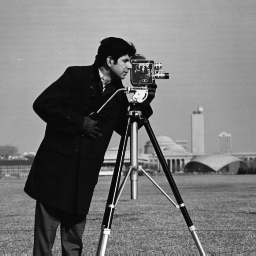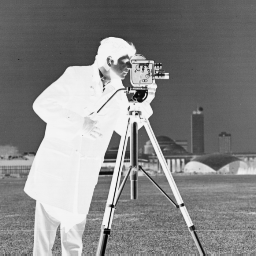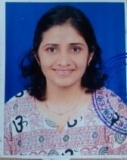# Point Processing in Image Processing using Python-OpenCV

OpenCV is the huge open-source library for computer vision, machine learning, and image processing and now it plays a major role in real-time operation which is very important in today’s systems. By using it, one can process images and videos to identify objects, faces, or even the handwriting of a human.

## Point processing in spatial domain

All the processing done on the pixel values. Point processing operations take the form –

`s = T ( r )`

Here, T is referred to as a grey level transformation function or a point processing operation, s refers to the processed image pixel value and r refers to the original image pixel value.

Image Negative:

`s = (L-1) – r, where L= number of grey levels`

Thresholding:

```s = L-1 for r > threshold
s = 0 for r < threshold```

Grey level slicing with background:

```s = L-1 for a < r < b,
here a and b define some specific range of grey level
s = r otherwise.```

Below is the implementation.

Original Input Image :`import` `cv2 ` `import` `numpy as np ` ` `  `  `  `# Image negative ` `img ``=` `cv2.imread(``'food.jpeg'``,``0``)  ` ` `  `# To ascertain total numbers of  ` `# rows and columns of the image, ` `# size of the image ` `m,n ``=` `img.shape ` `  `  `# To find the maximum grey level ` `# value in the image ` `L ``=` `img.``max``() ` `  `  `# Maximum grey level value  minus  ` `# the original image gives the ` `# negative image ` `img_neg ``=` `L``-``img ` `  `  `# convert the np array img_neg to  ` `# a png image ` `cv2.imwrite(``'Cameraman_Negative.png'``, img_neg) ` `  `  `# Thresholding without background  ` `# Let threshold =T ` `# Let pixel value in the original be denoted by r ` `# Let pixel value in the new image be denoted by s ` `# If rT, s=255 ` `  `  `T ``=` `150` ` `  `# create a array of zeros ` `img_thresh ``=` `np.zeros((m,n), dtype ``=` `int``)  ` `  `  `for` `i ``in` `range``(m): ` `     `  `    ``for` `j ``in` `range``(n): ` `         `  `        ``if` `img[i,j] <  T:  ` `            ``img_thresh[i,j]``=` `0` `        ``else``: ` `            ``img_thresh[i,j] ``=` `255` `  `  `  `  `# Convert array to png image ` `cv2.imwrite(``'Cameraman_Thresh.png'``, img_thresh) ` `  `  `# the lower threshold value ` `T1 ``=` `100` ` `  `# the upper threshold value ` `T2 ``=` `180`  ` `  `# create a array of zeros ` `img_thresh_back ``=` `np.zeros((m,n), dtype ``=` `int``) ` `  `  `for` `i ``in` `range``(m): ` `     `  `    ``for` `j ``in` `range``(n): ` `         `  `        ``if` `T1 < img[i,j] < T2:  ` `            ``img_thresh_back[i,j]``=` `255` `        ``else``: ` `            ``img_thresh_back[i,j] ``=` `img[i,j] ` ` `  `# Convert array to  png image ` `cv2.imwrite(``'Cameraman_Thresh_Back.png'``, img_thresh_back) `

Output : Image NegativeOutput : Image with Thresholding :Output : Image with Grey Level Slicing with BackgroundAttention geek! Strengthen your foundations with the Python Programming Foundation Course and learn the basics.

To begin with, your interview preparations Enhance your Data Structures concepts with the Python DS Course.

My Personal Notes arrow_drop_upAmi Munshi is an Asst Professor with MPSTME, Mumbai (NMIMS University) with the Electronics and Telecommunications specialization Focus areas on GfG Application of Python3 libs for Data/Image compression, Encryption, Data Science and Analytics applications

If you like GeeksforGeeks and would like to contribute, you can also write an article using contribute.geeksforgeeks.org or mail your article to contribute@geeksforgeeks.org. See your article appearing on the GeeksforGeeks main page and help other Geeks.

Please Improve this article if you find anything incorrect by clicking on the "Improve Article" button below.

Article Tags :

Be the First to upvote.

Please write to us at contribute@geeksforgeeks.org to report any issue with the above content.